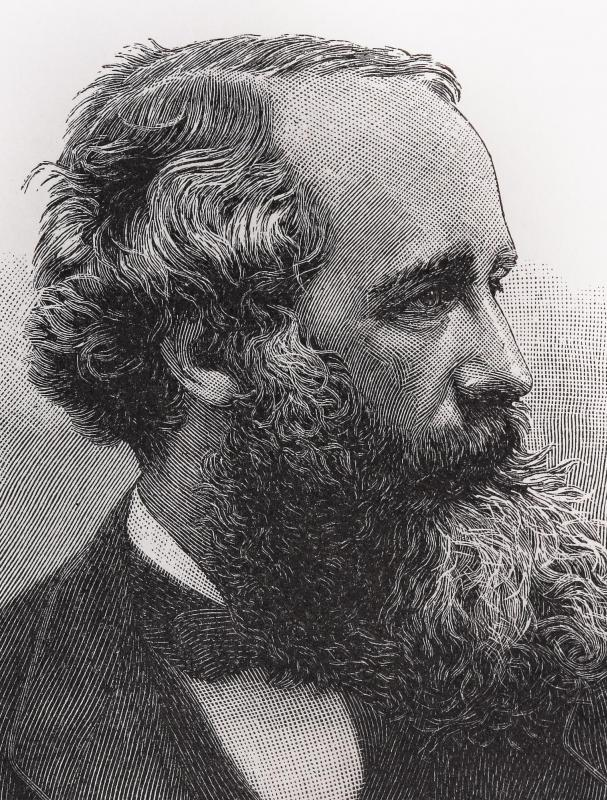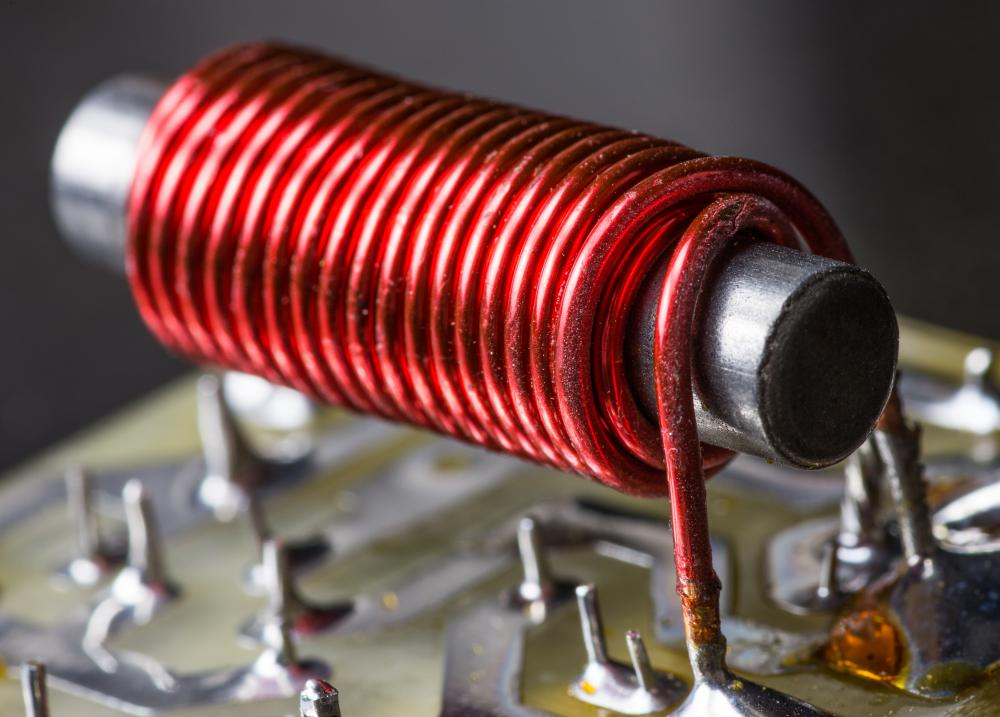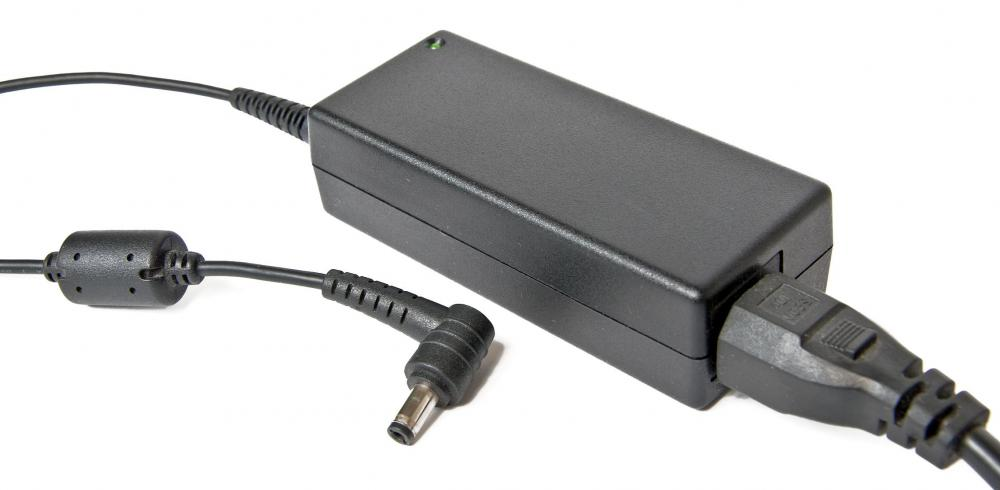# What is an Induced Current?

D.R. Satori
D.R. SatoriPhysicist James Clerk Maxwell wrote an equation that describes induced current.

A closed electrical circuit with energy flowing illustrates the two parts of the electromagnetic force: electricity and magnetism. Electricity is produced as electrons are pushed through a conducting wire by a source of voltage — a battery, for example. Not only does the original wire possess a flow of electrons, it also produces a magnetic field around that flow. These behaviors of electromagnetic energy come paired: the flow of electrons and the magnetic field that the flow creates. If one wire in which electrons flow is brought close to another wire, the magnetic field of the first wire will induce a flow — an induced current — along the second wire.An induced current can be created using an electromagnet and a magnet to manipulate the direction of the current.

In 1831, Michael Faraday published his discovery that a current in one wire could induce current in another wire. In 1862, this phenomenon of induced current was described mathematically by James Clerk Maxwell; it was based on the equations of colleagues who had described other energy exchanges, such as stress flows in solids and fluid flows in liquids. Maxwell’s equation illuminates the reasons for induced current, or inductance, by showing that the flow of electricity can be measured in two ways: as a voltage drop that forces electron flow and as a magnetic flow field, originating in the flow.Electrical transformers are commonly used to reduce electricity flow to devices such as laptops.

Induced current can be amplified when an electrically conducting wire is forced into a tight coil in the direction of a current. A transformer works by placing coils from two circuits parallel and close to one another so that electrical energy is transferred from one circuit to the next. This inductive coupling occurs when the magnetic fields emanating from the coils intersect in phase with one another, transferring a maximum amount of energy. This exchange is similar to the push given a child on a swing: if the push is timed properly, the swing is propelled upward at the maximum speed.

When a wire with a current is coiled about an iron rod, it can produce a magnetic field that can attract or repel the magnetic field of another such electromagnet. A motor and a generator each consist of two magnets, one moving and one fixed. The moving magnet, when it contacts the stationary magnet, induces a change in direction of electron flow, which results in the magnets repelling one another. This change in direction of induced current creates an alternating push and pull, causing the moving magnet to rotate. Inductance can work in the opposite direction when mechanical energy from a rotating propeller attached to a generator magnet forces the flow of electrons into storage batteries.

## You might also Like## Discuss this Article

Forgot password?
###### Register:
•Physicist James Clerk Maxwell wrote an equation that describes induced current.
•An induced current can be created using an electromagnet and a magnet to manipulate the direction of the current.
•Electrical transformers are commonly used to reduce electricity flow to devices such as laptops.